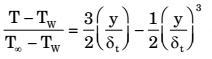## Heat Transfer Miscellaneous

#### Heat-Transfer

1. The ratios of the laminar hydrodynamic boundary layer thickness to thermal boundary layer thickness of flows of two fluids P and Q on a flat plate are 1/2 and 2 respectively. The Reynolds number based on the plate length for both the flows is 104. The Prandtl and Nusselt numbers for P are 1/8 and 35 respectively. The Prandlt and Nusselt numbers for Q are respectively

1.  δt = 1 × Pr-1/3 δ 1.026

 For fluid Q: 1/2 = δt = 1 × Pr-1/3 δ 1.026

⇒ Pr = 8
For fluid P: Laminar flow over flat plate Nu = 0.664 ReL1/2 Pr1/3 = 35
Similarly for fluid Q: Nu = 0.664 ReL1/2 Pr1/3
= 0.664 (104)1/2 81/3 ≃ 140

##### Correct Option: A

 δt = 1 × Pr-1/3 δ 1.026

 For fluid Q: 1/2 = δt = 1 × Pr-1/3 δ 1.026

⇒ Pr = 8
For fluid P: Laminar flow over flat plate Nu = 0.664 ReL1/2 Pr1/3 = 35
Similarly for fluid Q: Nu = 0.664 ReL1/2 Pr1/3
= 0.664 (104)1/2 81/3 ≃ 140

1. A pipe of 25 mm outer diameter carries steam. The heat transfer coefficient between the cylinder and surroundings is 5 W/m2K. It is proposed to reduce the heat loss from the pipe by adding insulation having a thermal conductivity of 0.05 W/mK. Which one of the following statements is TRUE?

 =kh

 =0.05m 5

(router) > rcritical
Thus, adding insulation shall decrease H.T. Rate.

##### Correct Option: C

 =kh

 =0.05m 5

(router) > rcritical
Thus, adding insulation shall decrease H.T. Rate.

1. For flow of fluid over a heated plate, the following fluid properties are known: viscosity = 0.001 Pa.s; specific heat at constant pressure = 1 kJ/kgK; thermal conductivity = 1 W/mK. The hydrodynamic boundary layer thickness at a specified location on the plate is 1 mm. The thermal boundary layer thickness at the same location is

1. Given:
µ =0.001 Pa – s
CP = 1 kJ/kg K
Kf = 1W/mK (Fluid thermal conductivity)
Hydrodynamic boundary layer thickness, δ = 1 mm

 Pr = μCP = 0.001 × 1000 = 1 Kf 1

δ t = δ (Cr)-1/3
= 1 × (1)-1/3 = 1 mm

##### Correct Option: C

Given:
µ =0.001 Pa – s
CP = 1 kJ/kg K
Kf = 1W/mK (Fluid thermal conductivity)
Hydrodynamic boundary layer thickness, δ = 1 mm

 Pr = μCP = 0.001 × 1000 = 1 Kf 1

δ t = δ (Cr)-1/3
= 1 × (1)-1/3 = 1 mm

1. The temperature distribution within the thermal boundary layer over a heated isothermal flat plate is given bywhere TW and T are the temperatures of plate and free stream respectively, and y is the normal distance measured from the plate. The local Nusselt number based on the thermal boundary layer thickness δt is given by

1. Only (b) satisfies the conditions

##### Correct Option: B

Only (b) satisfies the conditions

1. For laminar forced convection over a flat plate, if the free stream velocity increases by a factor of 2, the average heat transfer coefficient

1. For laminar flow, Nu = 0.664 (Re)0.5 (Pr)0.33

 hL = 0.664ρVD0.5 (Pr)0.23 k μ

h ∝ V0.5; h ∝ √V
So when free stream velocity increases by a fact or of 2, t hen t he aver age heat transfer coefficient rises by a factor of √2.

##### Correct Option: C

For laminar flow, Nu = 0.664 (Re)0.5 (Pr)0.33

 hL = 0.664ρVD0.5 (Pr)0.23 k μ

h ∝ V0.5; h ∝ √V
So when free stream velocity increases by a fact or of 2, t hen t he aver age heat transfer coefficient rises by a factor of √2.